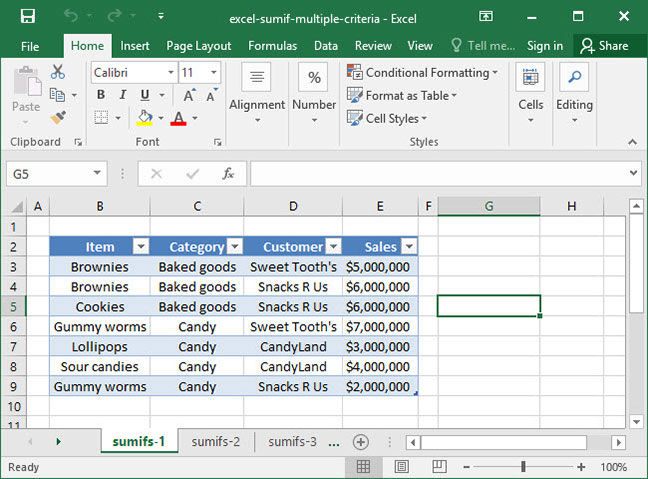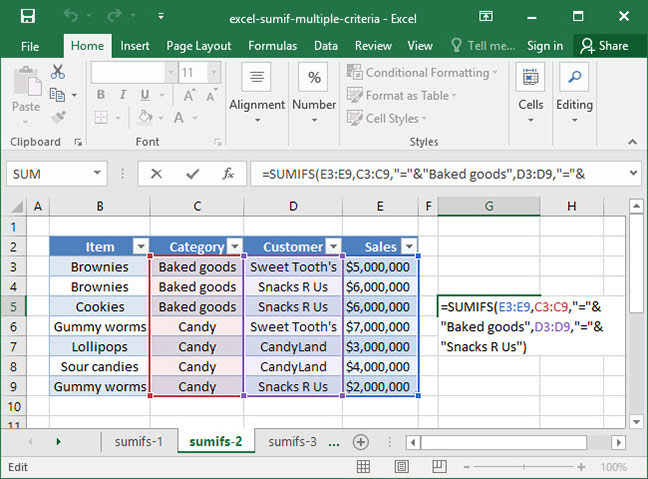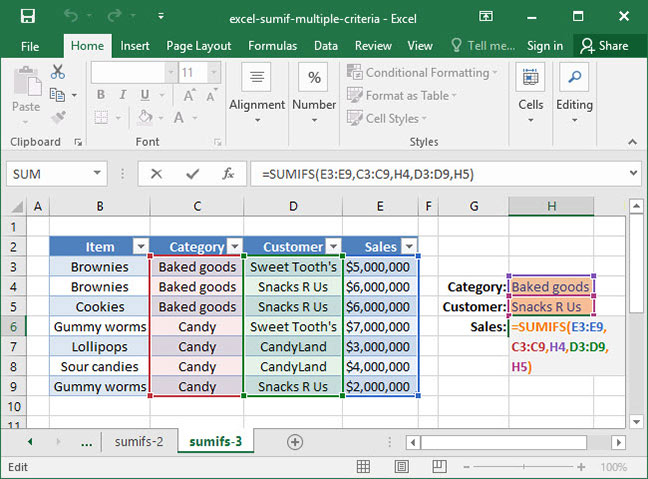# Excel's SUMIF with multiple criteria: SUMIFS

If you're a frequent user of Excel's SUMIF function, you may be wondering whether it's possible to perform a SUMIF across multiple criteria rather than a single criteria row. As it turns out, this is a very easy thing to do using Excel's SUMIFS function.

Let's say, for example, that a metal manufacturer has a table containing sales numbers by customer, product, and month. Using SUMIFS, they could compute the total amount of steel sold in January, or they could compute the total amount of iron sold to a particular customer across all months. The possibilities are endless — read on to find out how to use the function.

Knowledge of Excel's SUMIF, formula is required before you take this tutorial. if you're not yet comfortable with it, be sure to check out our SUMIF tutorial before reading on.

## Defining the problem

Take a look at the following spreadsheet, which lists SnackWorld's sales by item, category, and customer.Let's say we wanted to sum up SnackWorld's sales within a particular category (say, baked goods) to a particular customer (for example, Snacks R Us). We wouldn't be able to do this with SUMIF, because Category and Customer are two separate criteria columns. That's where SUMIFS comes in.

## Using SUMIFS

The basic syntax of the SUMIFS function is as follows:

`=SUMIFS(sum_range, criteria_range_1, criteria_1, criteria_range_2, criteria_2...)`

Note a couple key differences from the SUMIF function: first of all, our sum_range is the first argument provided, not the last one. Secondly, there are multiple criteria_range and criteria arguments tacked on to the end of the function. We can add as many of these argument as we'd like, as long as we do so in pairs: each criteria_range needs to have a corresponding criteria specified or the function will return an error.

Let's take a look at SUMIFS using the example outlined above with SnackWorld sales by customer. Here's a formula that calculates sales to Snacks R Us in the Baked Goods category:`=SUMIFS(E3:E9, C3:C9, =&"Baked Goods", D3:D9, =&"Snacks R Us")Output: \$12,000,000`

First, the above formula takes a look at the E3:E9 range, which it knows it has to take the SUM of. But, there are multiple criteria: the value in the C3:C9 range has to equal "Baked Goods", and the value in the D3:D9 range has to equal "Snacks R Us". There are only two cells that satisfy this criteria: cells E4 and E5, the sum of which is \$12,000,000.

## SUMIFS with a cell reference

We can also construct a SUMIFS formula with a cell reference. Let's modify the formula above to use dynamic cells rather than hard-coded values:`=SUMIFS(E3:E9, C3:C9, H4, D3:D9, H5)Output: \$12,000,000`

In the above example, we have replaced our criteria arguments with cell references to dynamic input cells that we've created on the sheet.

Also, remember from our SUMIF tutorial that when using the = sign as part of our criteria, you can just reference a cell directly and exclude the = entirely. That's what we've done in this example, replacing "="&H4 with a simple H4 and "="&H5 with H5.

## Using SUMIFS instead of SUMIF

Another interesting note on the above: It's possible to use SUMIFS with just one criteria pair, essentially converting it into a SUMIF function. In this situation, there's really no reason to choose one over the other — but we almost always use SUMIFS exclusively, even in cases in which we only have one criteria, so that we can remember the syntax more easily.

There you have it — sums across multiple criteria using the SUMIFS formula!

## Save an hour of work a day with these 5 advanced Excel tricks

Work smarter, not harder. Sign up for our 5-day mini-course to receive must-learn lessons on getting Excel to do your work for you.

• How to create beautiful table formatting instantly...
• Why to rethink the way you do VLOOKUPs...
• Plus, we'll reveal why you shouldn't use PivotTables and what to use instead...